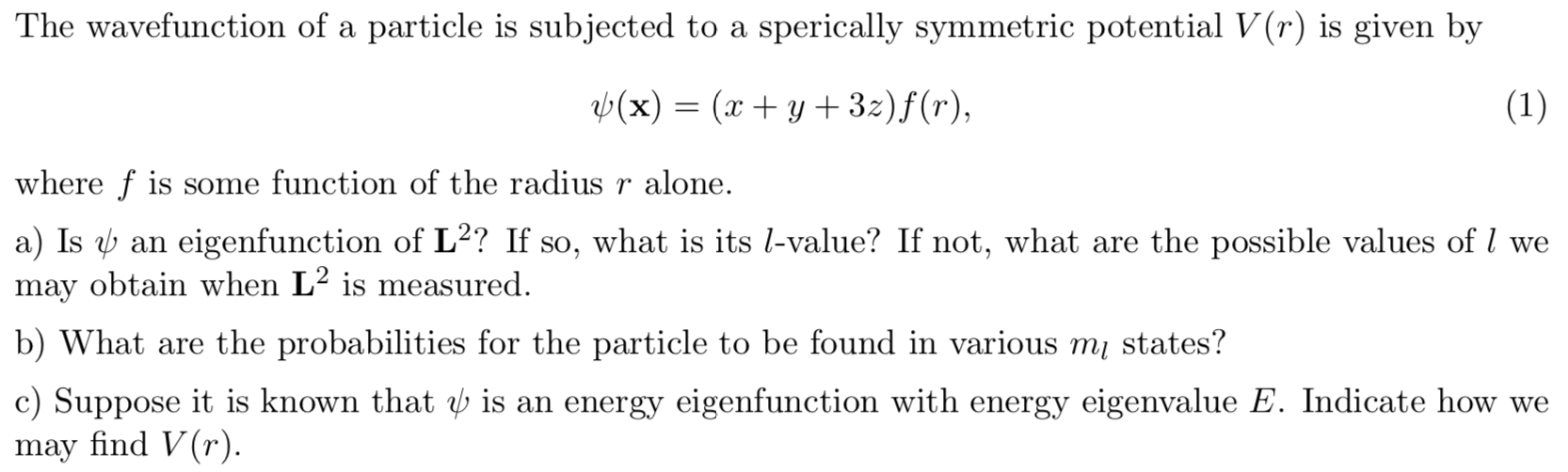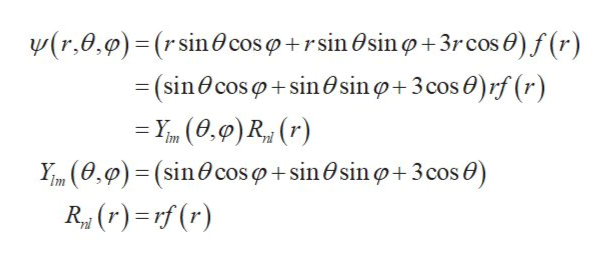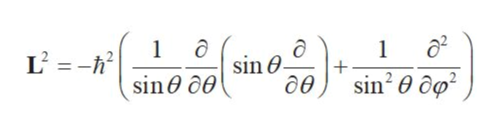# The wavefunction of a particle is subjected to a sperically symmetric potential V(r) is given byb(x)= (xy 32)f(r),(1)where f is some function of the radius r alone.a) Is an eigenfunction of L2? If so, what is its l-value? If not, what are the possible values of / wemay obtain when L2 is measured.b) What are the probabilities for the particle to be found in various m states?c) Suppose it is known that b is an energy eigenfunction with energy eigenvalue E. Indicate how wemay find V(r).

Question
20 views

It's a quantum mechanics question.help_outlineImage TranscriptioncloseThe wavefunction of a particle is subjected to a sperically symmetric potential V(r) is given by b(x)= (xy 32)f(r), (1) where f is some function of the radius r alone. a) Is an eigenfunction of L2? If so, what is its l-value? If not, what are the possible values of / we may obtain when L2 is measured. b) What are the probabilities for the particle to be found in various m states? c) Suppose it is known that b is an energy eigenfunction with energy eigenvalue E. Indicate how we may find V(r). fullscreen
check_circle

Step 1

The relation between the Cartesian and spherical coordinates is given as,

Step 2

Here θ is the polar angle and φ is the azimuthal angle.

Use the spherical polar coordinates to rewrite the wave function ψ in terms of r, θ, φ as,help_outlineImage Transcriptionclosew(r,0,0)= (r sin@ coso+rsin Osin o+3rcos 0) f (r) = (sin0cos o + sin@sin o+ 3 cos 0)rf (r) = Y (0.9) R, (r) Ym (0,0) = (sin0cos o + sin O sin o+3cos 0) R, (r) =rf (r) fullscreen
Step 3

Here Ylm represents spherical harmonics and Rnl (r) represents the radial part of the wave function.

a)

The operator...help_outlineImage Transcriptionclose1ô sin@. L' = -h? ( sin@ col 0 0 sin? 0 ôg² дө fullscreen

### Want to see the full answer?

See Solution

#### Want to see this answer and more?

Solutions are written by subject experts who are available 24/7. Questions are typically answered within 1 hour.*

See Solution
*Response times may vary by subject and question.
Tagged in

### Science